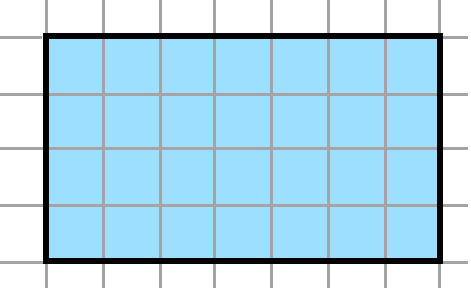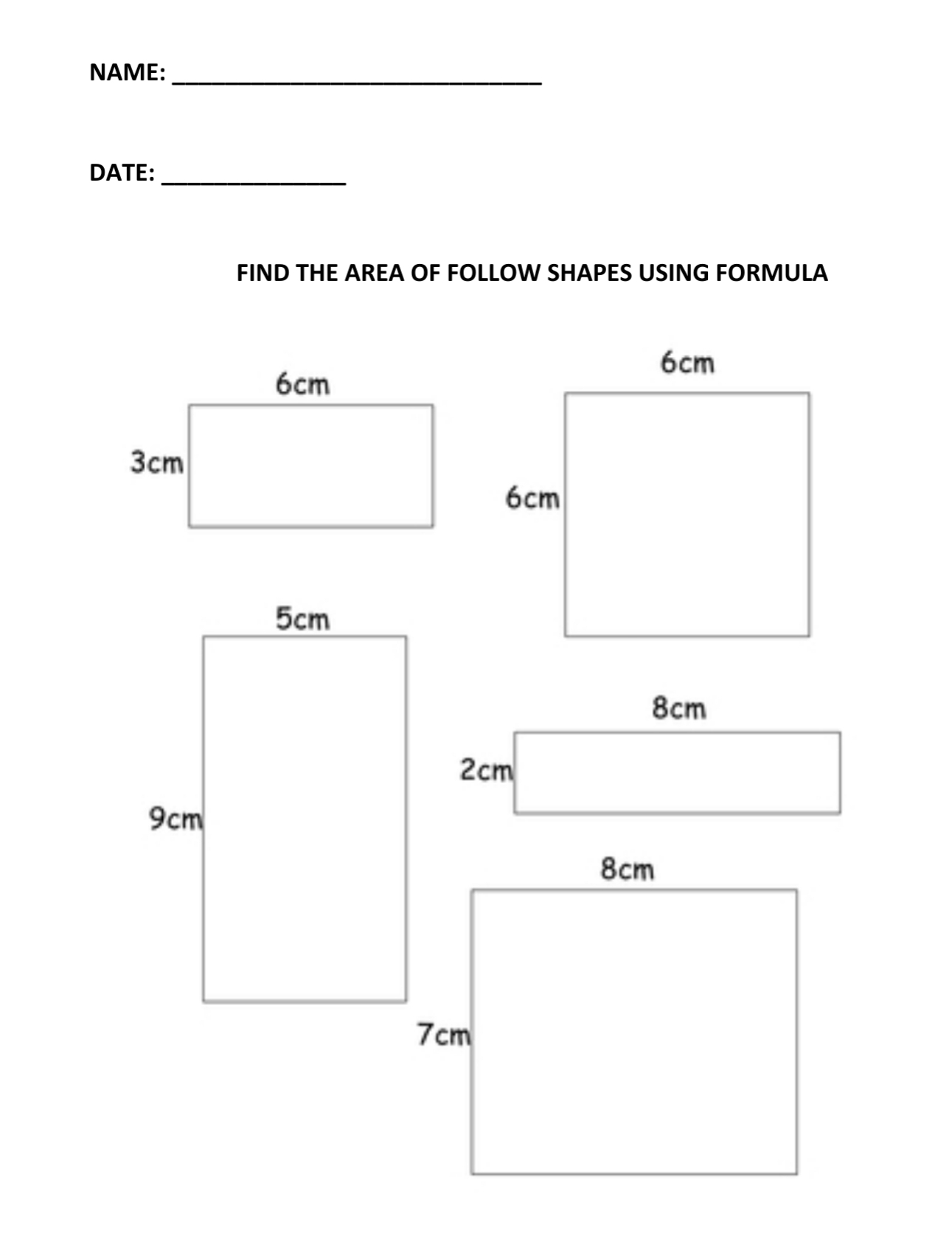# Introduction to Area of Squares and Rectangles by Counting and Using Formula | Weekly Assessment (Test) Primary 3 (Basic 3) – Mathematics

### MATHEMATICS

THIRD TERM

WEEK 3

PRIMARY 3

THEME – PRIMARY MEASUREMENT

PREVIOUS LESSON – Length – Non Standard Measurements (Compare Arms and Foot Length) | Standard Measurements in Metres and Centimetres | Conversion of Metres to Centimetres and Vice Versa Primary 3 (Basic 3) – Mathematics

### LEARNING AREA

1. Introductory Activities

2. Area of Squares and Rectangles by Counting

3. Area of Squares and Rectangles by Counting in cm²

4. Area of Squares and Rectangles Using Formula in cm²

5. Lesson Evaluation and Weekly Assessment (Test)

### PERFORMANCE OBJECTIVES

By the end of the lesson, most pupils should have attained the following objectives (cognitive, affective and psychomotor) and should be able to –

1. find the area of rectangles and squares by counting the number of squares they cover.

2. find the area of rectangles and squares by counting the number of square centimetres.

3. find the area of rectangles and squares, using the formula.

### RATIONALE

Area is the size of a surface. The most common surfaces within and around us are either square or rectangular surfaces. Objects with either of these surfaces occupied certain portion of available space.

The concept of area of squares and rectangles are very important in arrangements and allocation of space. For example, the size of school building and classroom.

This lesson will enable the pupils to recognise and appreciate the importance of areas.

### ENTRY BEHAVIOUR

The pupils can count number of objects in a container.

### INSTRUCTIONAL MATERIALS

The teacher will teach the lesson with the aid of:

1. Square and Rectangular shapes

2. Writing materials

### METHOD OF TEACHING

Choose a suitable and appropriate methods for the lessons.

Note – Irrespective of choosing methods of teaching, always introduce an activities that will arouse pupil’s interest or lead them to the lessons.

### REFERENCE MATERIALS

1. Scheme of Work

2. 9 – Years Basic Education Curriculum

3. Course Book

4. All Relevant Material

5. Online Information

### WRITING INSTRUCTION

Copy as I write or draw as I write. This instruction should be given when you need the pupils to write or draw.

### LESSON 1 – INTRODUCTORY ACTIVITIES

Teacher’s Activities – Based on the previous group, give each group square and rectangular shapes for them to examine, identify, compare, describe and state the difference between the two (2) shapes.

Cold call anyone in the group for presentation.

Pupil’s Activities – Each shape has four (4) lengths. Square shape has all lengths equal. While rectangular shape has two (2) opposite lengths equal.

Teacher’s Remark – Correct, square and rectangle are four (4) sided shaped. Today, we are going to the area of square and rectangle.

### GROUP WORK

You can regroup the pupils and give each group the following set of instructions –

1. Examine the following shapes and indicate each name.2. Find the area of rectangles and squares by counting the number of squares they cover.

3. What is area?

Teacher’s Activities – Cold call for group work presentation.

Teacher’s remarks – Good job you all for active participation. Area is the size of a surface or space occupied by the surface of an object.

### LESSON EVALUATION### LESSON 2 – INTRODUCTORY ACTIVITIES

Teacher’s Activities – Display chart showing cm² (centimeters square) for the pupils to pronounce properly and write correctly.

If the number of the class is not too large, allow the pupils pronounce correctly. But if they are much, scan and be sure that they are participating.

Pupil’s Activities/Expected Response – Pronounce and write cm².

Teacher’s Remark – Centimetres square is the standard unit for area squares and rectangles. Today, we are going find the area of squares and rectangles by counting and writing in centimetres square.

### AREA OF SQUARES AND RECTANGLES BY COUNTING IN CENTIMETRES SQUARE

INDIVIDUAL WORK

As in their previous lesson evaluation, ask the pupils to write the areas in cm².

### LESSON 3 – INTRODUCTORY ACTIVITIES

Teacher’s Activities – Ask the pupils to the following numbers on the chart or board –

If cm – centimetres and cm² – centimetres square, read –

2 cm, 4 cm, 5 cm, 7cm, 10 cm², 12 cm², 16 cm².and 25 cm²

Pupil’s Activities/Expected Responses – Two centimetres, four centimetres, 5 centimetres, 7 centimetres, 10 centimetres square, 12 centimetres square, 26 centimeters square and 25 centimetres square.

Teacher’s Remark – Correct, thumb up for you all. The standard unit of each length of the square and rectangle are in cm while the area are in cm². Today, we are going find the area of square and rectangle in cm and cm² by counting and multiplication cm².

### GROUP WORK

Guided instructions,

1. The shape below has four (4) lengths. Name all the lengths as A, B, C and D.2. Count the number of squared shapes on A, B, C and D. And record answers.

Expected response – A and C – 7 cm and B and D – 4 cm.

3. Multiple A by D or C by D and record your answer in cm².

For example, 7 cm x 4 cm = 28 cm².

4. Find the area of the shape by counting and record your answer in cm².

Expected response – 28 cm².

Expected response – The answers are the same.

Teacher’s Activities – Cold call for group work presentation. Thumb up for each and everyone of you for active participation.

Teacher’s Remarks – We can find the areas of square and rectangle by counting the square units or multiple the unit lengths together. That’s length and breadth – L X B. While for L X L for square.

LESSON EVALUATION

Find the area of rectangles and squares, using the formula.### REVISION AND WEEKLY ASSESSMENT

As stated in performance objectives or lesson evaluation.

### PRESENTATION

To deliver the lesson, the teacher adopts the following steps:

1. To introduce the lesson, the teacher revises the previous lesson. Based on this, he/she asks the pupils some questions;

2. Guides pupils to find the pupils to find the area of squares and rectangles by counting and using formula in cm².

Pupil’s Activities – Follow the teacher’s instructions to find the area of squares and rectangles by counting and using formula in cm².

### CONCLUSION

To conclude the lesson for the week, the teacher revises the entire lesson and links it to the following week’s lesson.

### LESSON EVALUATION

1. find the area of rectangles and squares by counting the number of squares they cover.

2. find the area of rectangles and squares by counting the number of square centimetres.

3. find the area of rectangles and squares, using the formula.

### ATTEMPT ALL THE QUESTIONS

1. _____________ is the size of a surface.

A. Square

B. Area

C. Rectangle

2. _____________ has four (4) equal lengths.

A. Square

B. Area

C. Rectangle

3. _____________ has two (2) equal opposite lengths.

A. Square

B. Area

C. Rectangle

4. _____________ has four (4) lengths.

A. Square

B. Rectangle

C. Square and rectangle

5. _____________ is the standard unit of area for square and rectangle.

A. cm

B. cl²

C. cm²